# Physics 121 Electricity Magnetism Lecture 1 Dale E

• Slides: 32Physics 121: Electricity & Magnetism – Lecture 1 Dale E. Gary Wenda Cao NJIT Physics Department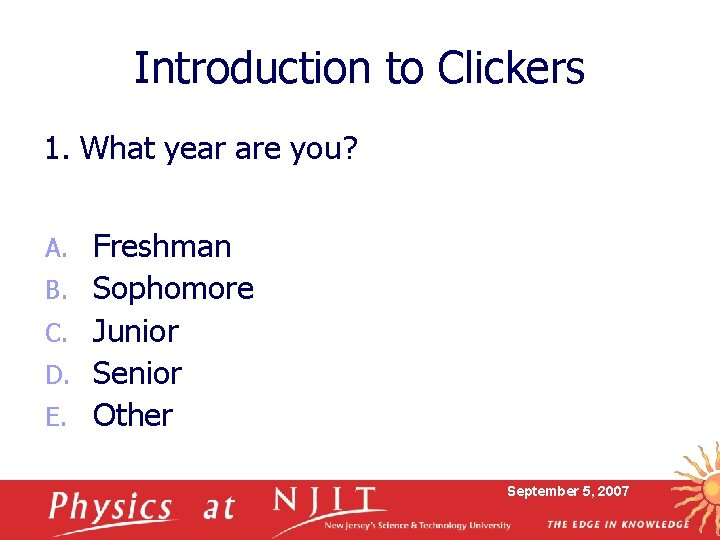Introduction to Clickers 1. What year are you? A. B. C. D. E. Freshman Sophomore Junior Senior Other September 5, 2007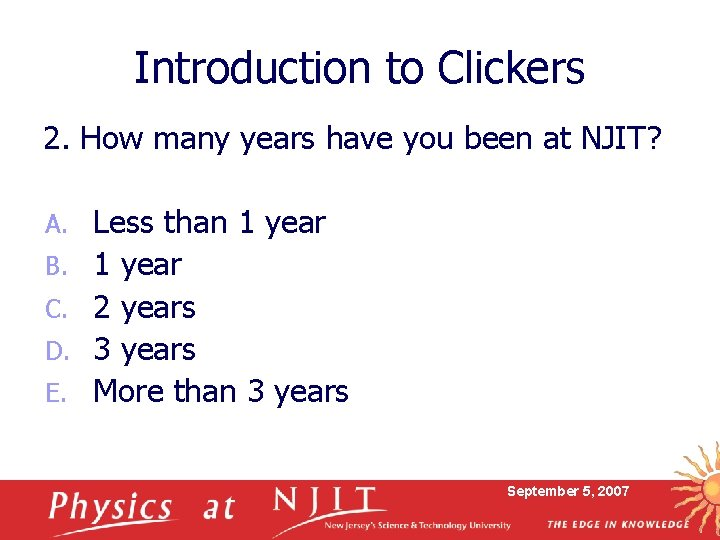Introduction to Clickers 2. How many years have you been at NJIT? A. B. C. D. E. Less than 1 year 2 years 3 years More than 3 years September 5, 2007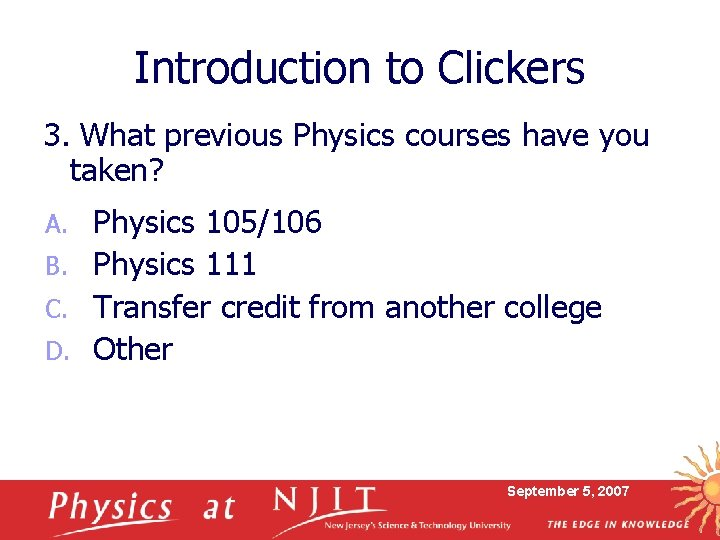Introduction to Clickers 3. What previous Physics courses have you taken? Physics 105/106 B. Physics 111 C. Transfer credit from another college D. Other A. September 5, 2007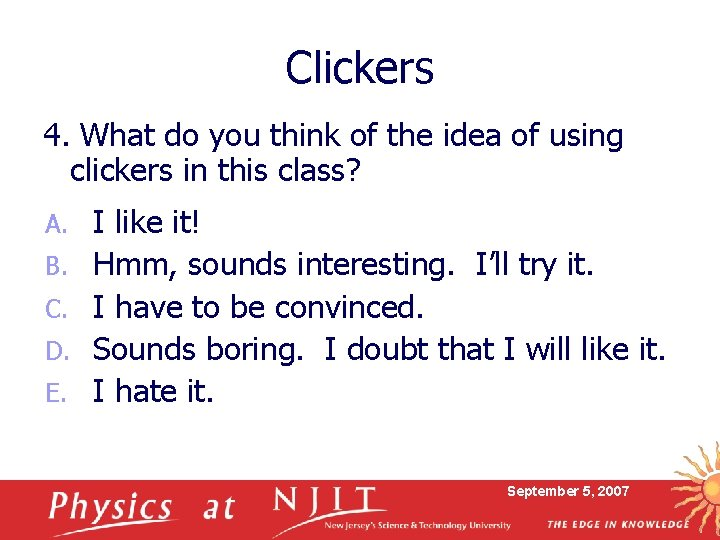Clickers 4. What do you think of the idea of using clickers in this class? A. B. C. D. E. I like it! Hmm, sounds interesting. I’ll try it. I have to be convinced. Sounds boring. I doubt that I will like it. I hate it. September 5, 2007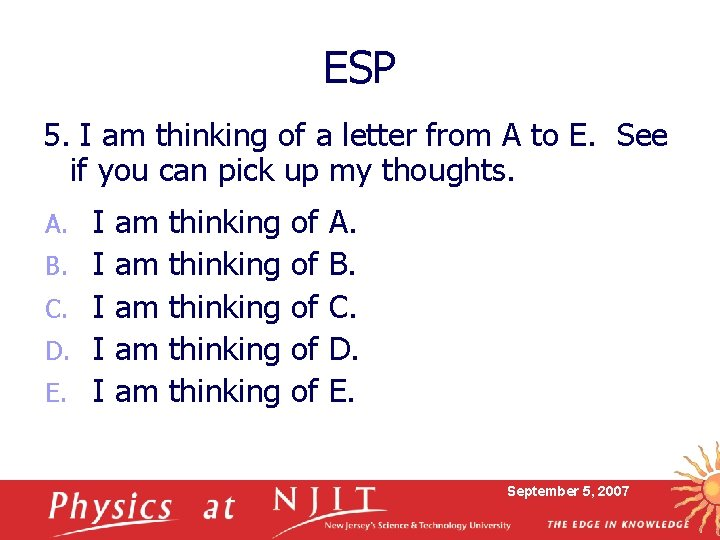ESP 5. I am thinking of a letter from A to E. See if you can pick up my thoughts. A. B. C. D. E. I I I am am am thinking thinking of of of A. B. C. D. E. September 5, 2007ESP 2 6. Let’s do it again, but show the graph while you select your answer. I am thinking of a letter from A to E. What letter am I thinking of? A. B. C. D. E. I I I am am am thinking thinking of of of A. B. C. D. E. September 5, 2007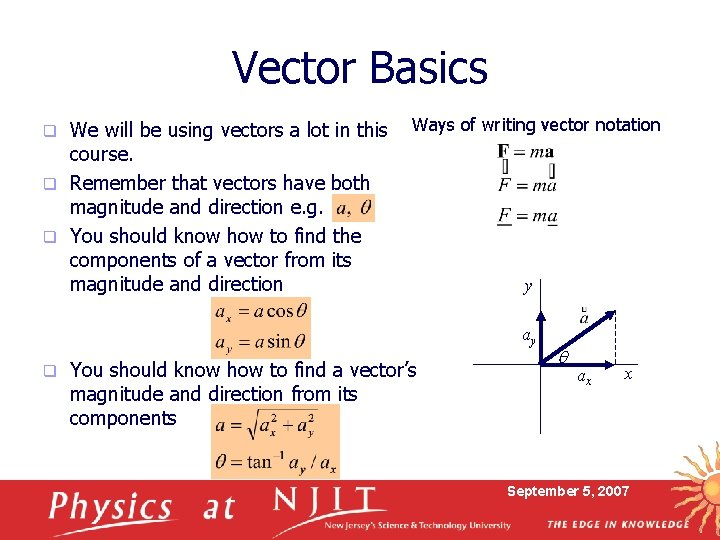Vector Basics We will be using vectors a lot in this course. q Remember that vectors have both magnitude and direction e. g. q You should know how to find the components of a vector from its magnitude and direction q Ways of writing vector notation y ay q You should know how to find a vector’s magnitude and direction from its components q ax x September 5, 2007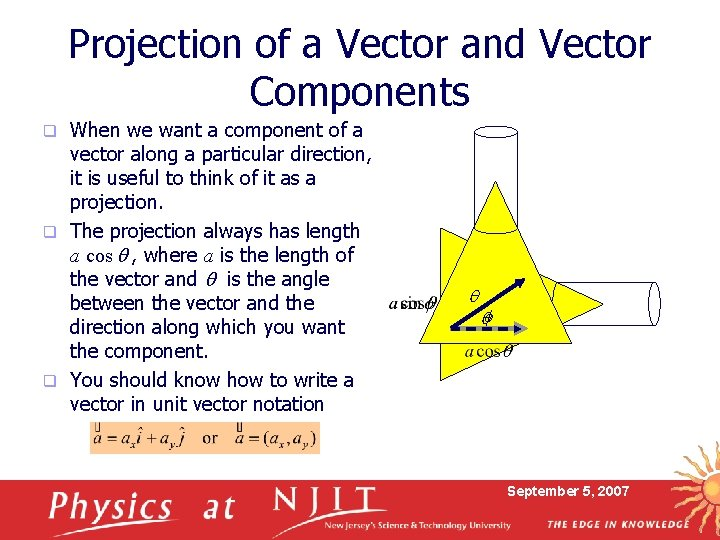Projection of a Vector and Vector Components When we want a component of a vector along a particular direction, it is useful to think of it as a projection. q The projection always has length a cos q , where a is the length of the vector and q is the angle between the vector and the direction along which you want the component. q You should know how to write a vector in unit vector notation q q qf September 5, 2007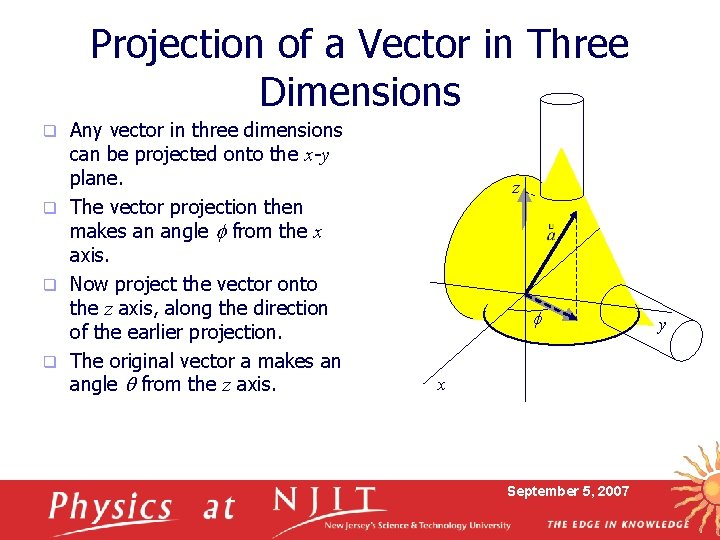Projection of a Vector in Three Dimensions Any vector in three dimensions can be projected onto the x-y plane. q The vector projection then makes an angle f from the x axis. q Now project the vector onto the z axis, along the direction of the earlier projection. q The original vector a makes an angle q from the z axis. q z q f x September 5, 2007 yVector Basics You should know how to generalize the case of a 2 -d vector to three dimensions, e. g. 1 magnitude and 2 directions q Conversion to x, y, z components q z q q Conversion from x, y, z components f x q Unit vector notation: September 5, 2007 y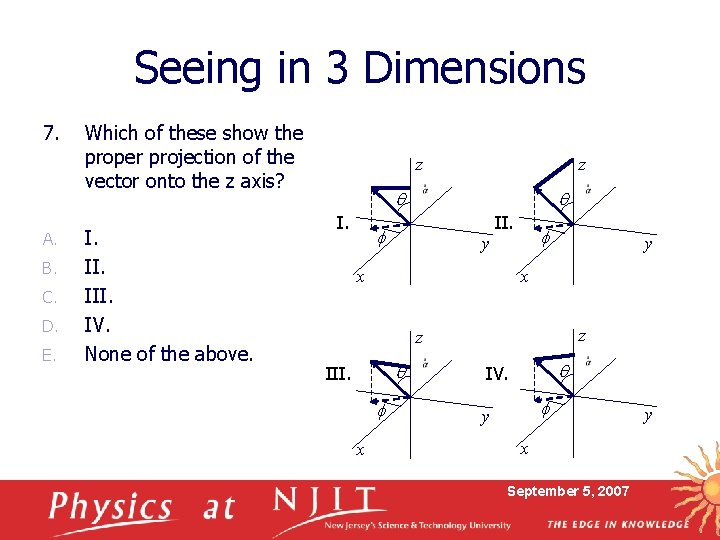Seeing in 3 Dimensions 7. A. B. C. D. E. Which of these show the proper projection of the vector onto the z axis? I. III. IV. None of the above. z z q II. f f y x z z q III. f x q IV. f y x September 5, 2007 y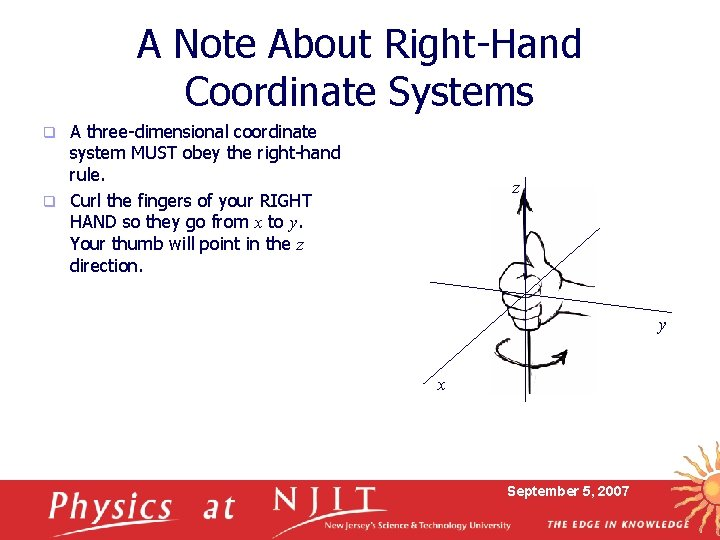A Note About Right-Hand Coordinate Systems A three-dimensional coordinate system MUST obey the right-hand rule. q Curl the fingers of your RIGHT HAND so they go from x to y. Your thumb will point in the z direction. q z y x September 5, 2007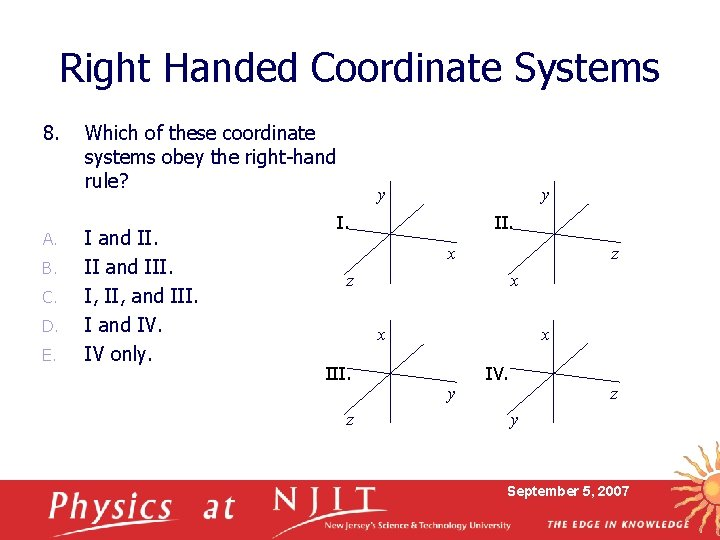Right Handed Coordinate Systems 8. A. B. C. D. E. Which of these coordinate systems obey the right-hand rule? I and II. II and III. I, II, and III. I and IV. IV only. y y I. II. x z z x x III. z x y IV. z y September 5, 2007Vector Math q Vector Inverse n q Vector Addition n q Use head-tail method, or parallelogram method Vector Subtraction n q Just switch direction Use inverse, then add Vector Multiplication n Two kinds! Scalar, or dot product Vector, or cross product Vector Addition by Components September 5, 2007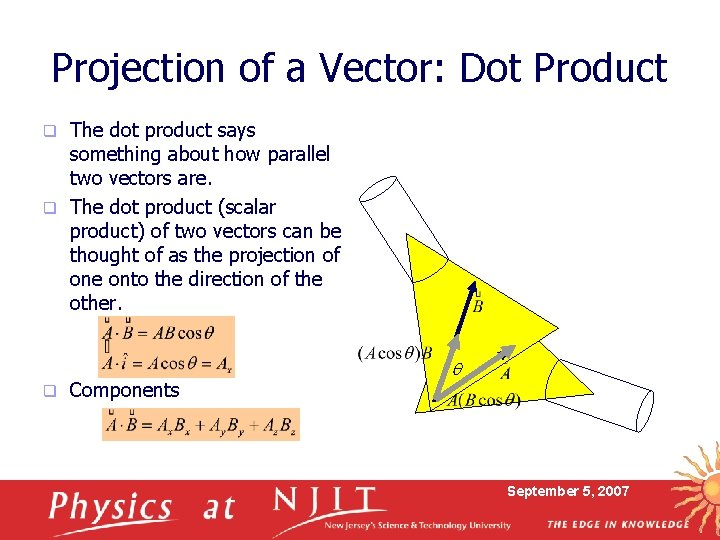Projection of a Vector: Dot Product The dot product says something about how parallel two vectors are. q The dot product (scalar product) of two vectors can be thought of as the projection of one onto the direction of the other. q q Components q September 5, 2007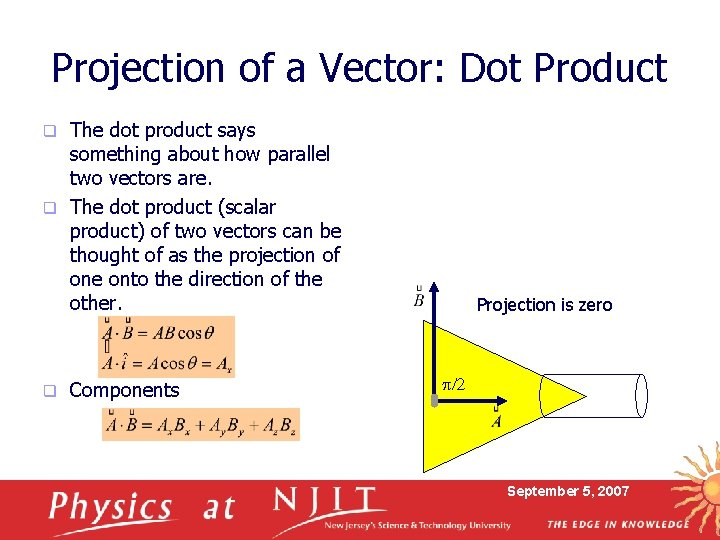Projection of a Vector: Dot Product The dot product says something about how parallel two vectors are. q The dot product (scalar product) of two vectors can be thought of as the projection of one onto the direction of the other. q q Components Projection is zero p/2 September 5, 2007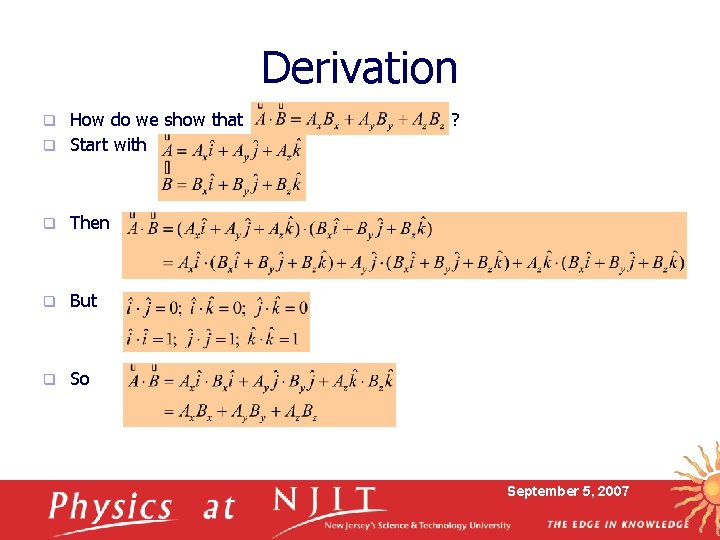Derivation How do we show that q Start with q q Then q But q So ? September 5, 2007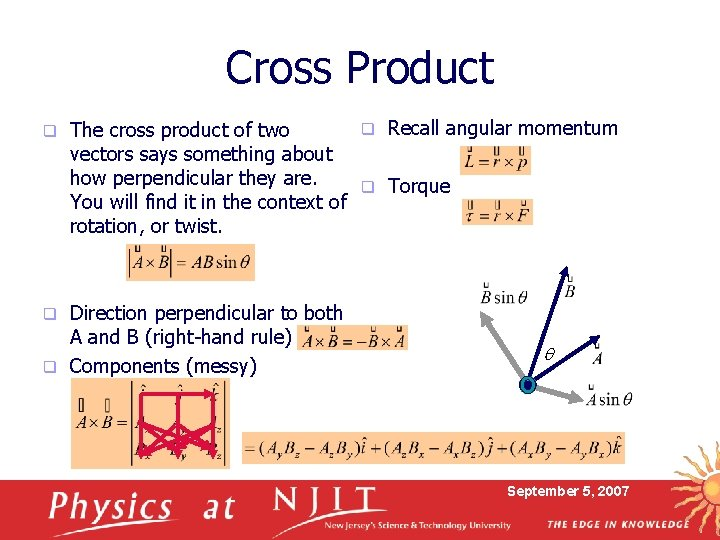Cross Product q q Recall angular momentum The cross product of two vectors says something about how perpendicular they are. q Torque You will find it in the context of rotation, or twist. Direction perpendicular to both A and B (right-hand rule) q Components (messy) q q September 5, 2007Derivation How do we show th at q Start with ? q q Then q But q So September 5, 2007Scalar Fields q A scalar field is just one where a quantity in “space” is represented by numbers, such as this temperature map. q Here is another scalar field, height of a mountain. Contours far apart Contours close together Contours steeper flatter Side View September 5, 2007Gradients and Gravity Height contours h, are proportional to potential energy U = mgh. If you move along a contour, your height does not change, so your potential energy does not change. q If you move downhill, on say a 6% grade, it means the slope is 6/100 (for every 100 m of horizontal motion, you move downward by 6 m). q q Grade and gradient mean the same thing. A 6% grade is a gradient of 6 100 F = mg sinq dl q dh mg September 5, 2007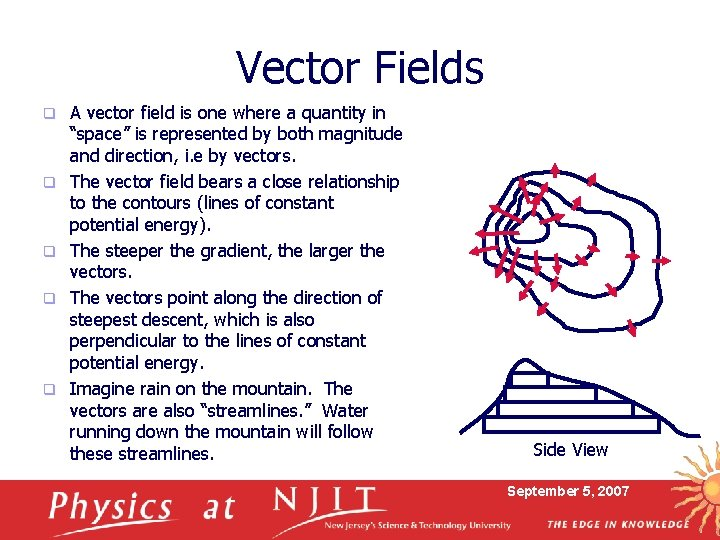Vector Fields q q q A vector field is one where a quantity in “space” is represented by both magnitude and direction, i. e by vectors. The vector field bears a close relationship to the contours (lines of constant potential energy). The steeper the gradient, the larger the vectors. The vectors point along the direction of steepest descent, which is also perpendicular to the lines of constant potential energy. Imagine rain on the mountain. The vectors are also “streamlines. ” Water running down the mountain will follow these streamlines. Side View September 5, 2007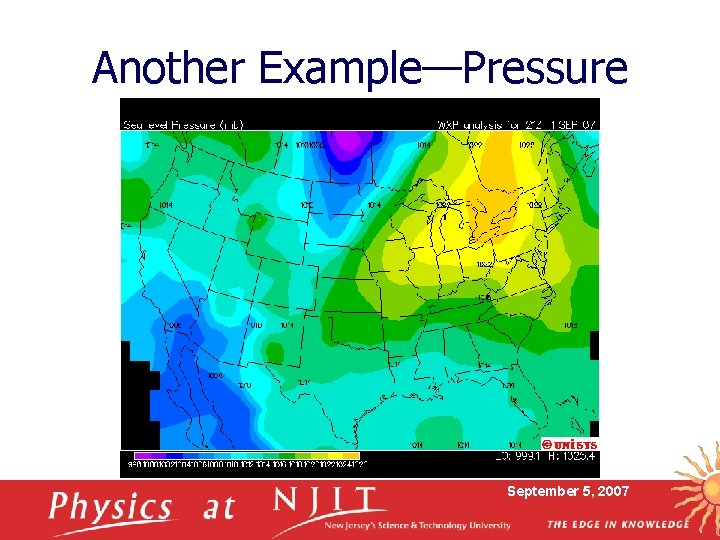Another Example—Pressure September 5, 2007Another Example—Pressure September 5, 2007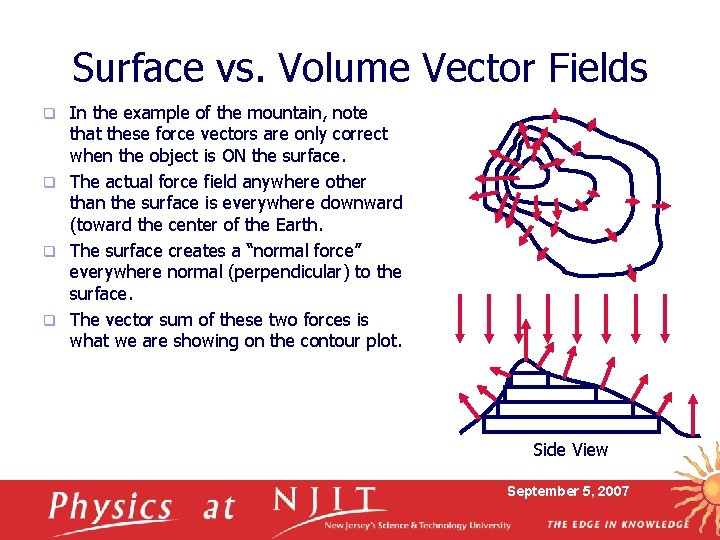Surface vs. Volume Vector Fields In the example of the mountain, note that these force vectors are only correct when the object is ON the surface. q The actual force field anywhere other than the surface is everywhere downward (toward the center of the Earth. q The surface creates a “normal force” everywhere normal (perpendicular) to the surface. q The vector sum of these two forces is what we are showing on the contour plot. q Side View September 5, 2007Vector Field Due to Gravity q When you consider the force of Earth’s gravity in space, it points everywhere in the direction of the center of the Earth. But remember that the strength is: q This is an example of an inverse-square force (proportional to the inverse square of the distance). September 5, 2007Idea of Test Mass q Notice that the actual amount of force depends on the mass, m: q It is convenient to ask what is the force per unit mass. The idea is to imagine putting a unit test mass near the Earth, and observe the effect on it: q g(r) is the “gravitational field. ” September 5, 2007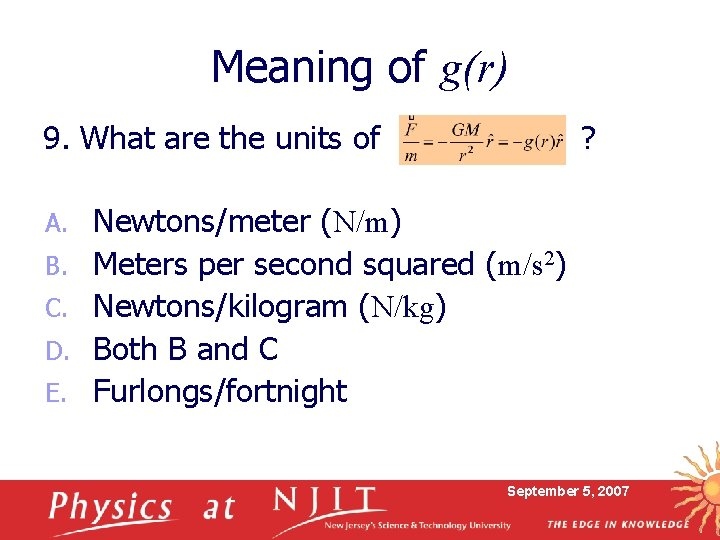Meaning of g(r) 9. What are the units of A. B. C. D. E. ? Newtons/meter (N/m) Meters per second squared (m/s 2) Newtons/kilogram (N/kg) Both B and C Furlongs/fortnight September 5, 2007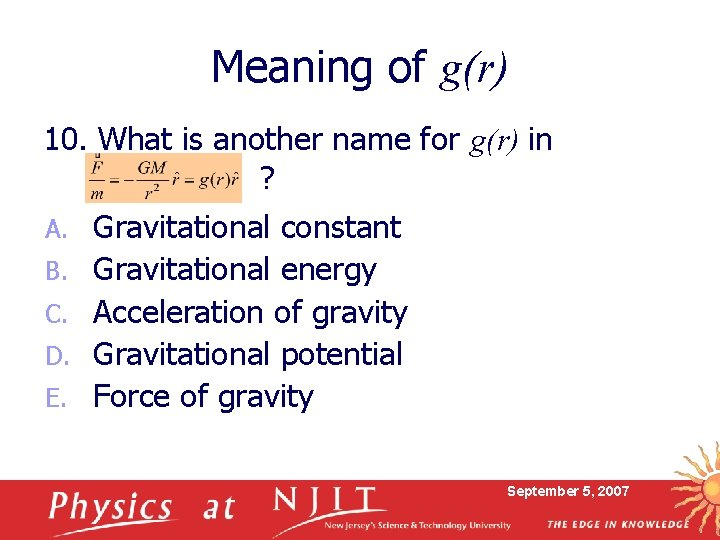Meaning of g(r) 10. What is another name for g(r) in ? A. B. C. D. E. Gravitational constant Gravitational energy Acceleration of gravity Gravitational potential Force of gravity September 5, 2007Gravitational Field We can therefore think of the “action-at-a-distance” of gravity as a field that permeates all of space. q We draw “field lines” that show both the direction and strength of the field (from the density of field lines). q The field cannot be seen or touched, and has no effect until you consider a second mass. q What happens if we have two equal masses? Superposition— just vector sum the two fields. q September 5, 2007Gravitational Field of Two Equal Masses Again, think of adding a small test mass. q The force vectors show the direction and strength of the force on such a test mass. q We can draw field lines that follow the force vectors. q We will be using these same concepts when we talk about electric charge in Lecture 2, and the electric field in Lecture 3. September 5, 2007